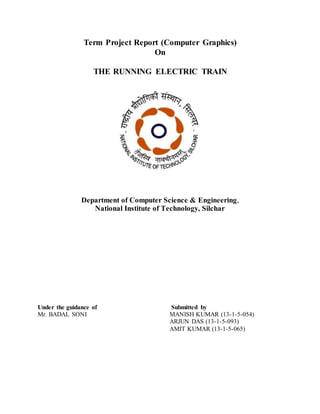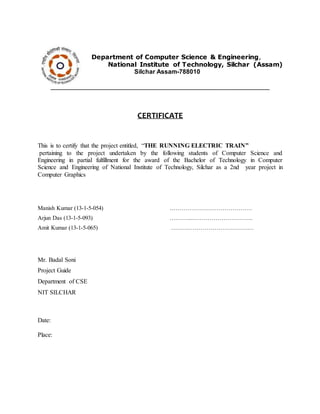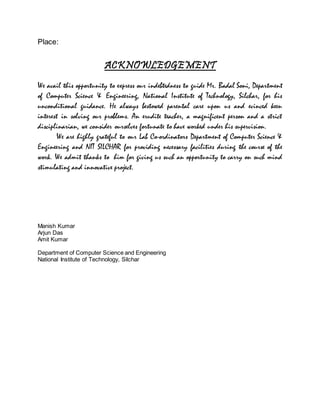Ce diaporama a bien été signalé.
Nous utilisons votre profil LinkedIn et vos données d’activité pour vous proposer des publicités personnalisées et pertinentes. Vous pouvez changer vos préférences de publicités à tout moment.Chargement dans…3
×
1 sur 19

Computer Graphics Project- The Running Train

15

Partager

An electric locomotive is a locomotive powered by electricity from overhead lines, a third rail or on-board energy storage such as a battery or fuel cell.

This project is all about modelling with many more features of openGL. The starting point is a small virtual space that consists of a grassy plain with a simple parallel railway track running on it. It also consists of a plain of clear sky. It also contains engine & bogies with electric wires for power supply. Lastly, it contains wheels rotating & translating in forward direction.

Tout voir

Computer Graphics Project- The Running Train

1. 1. Term Project Report (Computer Graphics) On THE RUNNING ELECTRIC TRAIN Department of Computer Science & Engineering, National Institute of Technology, Silchar Under the guidance of Submitted by Mr. BADAL SONI MANISH KUMAR (13-1-5-054) ARJUN DAS (13-1-5-093) AMIT KUMAR (13-1-5-065)
2. 2. Department of Computer Science & Engineering, National Institute of Technology, Silchar (Assam) Silchar Assam-788010 ______________________________________________________________ CERTIFICATE This is to certify that the project entitled, “THE RUNNING ELECTRIC TRAIN” pertaining to the project undertaken by the following students of Computer Science and Engineering in partial fulfillment for the award of the Bachelor of Technology in Computer Science and Engineering of National Institute of Technology, Silchar as a 2nd year project in Computer Graphics Manish Kumar (13-1-5-054) …………………………………… Arjun Das (13-1-5-093) ….……..…………………………. Amit Kumar (13-1-5-065) …………………………………… Mr. Badal Soni Project Guide Department of CSE NIT SILCHAR Date: Place:
3. 3. Place: ACKNOWLEDGEMENT We avail this opportunity to express our indebtedness to guide Mr. Badal Soni, Department of Computer Science & Engineering, National Institute of Technology, Silchar, for his unconditional guidance. He always bestowed parental care upon us and evinced keen interest in solving our problems. An erudite teacher, a magnificent person and a strict disciplinarian, we consider ourselves fortunate to have worked under his supervision. We are highly grateful to our Lab Co-ordinators Department of Computer Science & Engineering and NIT SILCHAR for providing necessary facilities during the course of the work. We admit thanks to him for giving us such an opportunity to carry on such mind stimulating and innovative project. Manish Kumar Arjun Das Amit Kumar Department of Computer Science and Engineering National Institute of Technology, Silchar
4. 4. Table of Contents 1. Introduction…………………………………………………….. 2. History of Electric train………………………………………... 3. Design and Implementation……………………………………… 4. Source Codeof the application………………………………….. 5. Simulation Result………………………………………………… 6. Conclusion………………………………………………………… 7. Reference and Bibliography……………………………………………
5. 5. Introduction An electric locomotive is a locomotive powered by electricity from overhead lines, a third rail or on-board energy storage such as a battery or fuel cell. Electric locomotives with on-board fuelled prime movers, such as diesel engines or gas turbines, are classed as diesel-electric or gas turbine-electric locomotives because the electric generator/motor combination serves only as a power transmission system. Electricity is used to eliminate smoke and take advantage of the high efficiency of electric motors, but the cost of electrification means that usually only heavily used lines can be electrified. This project is all about modelling with many more features of openGL. The starting point is a small virtual space that consists of a grassy plain with a simple parallel railway track running on it. It also consists of a plain of clear sky. It also contains engine & bogies with electric wires for power supply. Lastly, it contains wheels rotating & translating in forward direction.
6. 6. HISTORY of Electric Train The first known electric locomotive was built in 1837 by chemist Robert Davidson of Aberdeen. It was powered by galvanic cells (batteries). Davidson later built a larger locomotive named Galvani, exhibited at the Royal Scottish Society of Arts Exhibition in 1841. The seven-ton vehicle had two direct drive reluctance motors, with fixed electromagnets acting on iron bars attached to a wooden cylinder on each axle, and simple commutators. It hauled a load of six tons at four miles per hour for a distance of one and a half miles. It was tested on the Edinburgh and Glasgow railway in September of the following year, but the limited power from batteries prevented its general use. It was destroyed by railway workers, who saw it as a threat to their security of employment. The first electric passenger train was presented by Werner von Siemens at Berlin in 1879. The locomotive was driven by a 2.2 kW, series-wound motor, and the train, consisting of the locomotive and three cars, reached a speed of 13 km/h. During four months, the train carried 90,000 passengers on a 300-metre-long circular track. The electricity (150 V DC) was supplied through a third insulated rail between the tracks. A contact roller was used to collect the electricity. The world's first electric tram line opened in Lichterfelde near Berlin, Germany, in 1881. It was built by Werner von Siemens. Volk's electric railway opened in 1883 in Brighton . Also in 1883, Modling and Hinterbruhi Tram opened near Vienna in Austria. It was the first in the world in regular service powered from an overhead line. Five years later, in the U.S. electric trolleys were pioneered in 1888 on the Richmond Union Passenger Railway, using equipment designed by Frank J.Sprague.
7. 7. Design and Implementation Creating a Train in Computer graphics is not that much hard but logical. In this project we are going to implement an OpenGL Projects on the running electric train. The best method for building the objects is by placing the drawing code for each object in a separate function. Each object is defined using a coordinate system that makes modelling convenient. Sky, environment , track , electric wires , train (bogie , engine doors , windows , electrical stimulator) are made by using mode GL_POLYGON . Pantograph and Joints are done by GL_LINE_LOOP & GL_LINE mode. After we finish the drawing our next aim would be to give motion to the train. As we are going to draw a simple train so our track will be a straight not in zigzag manner. It is made of many boxes or rectangles while wheel is circular. The two parallel line have the electricity flow for the train running, we have electric engine here.
8. 8. Source code #include<stdio.h> #include<math.h> #include<GL/glut.h> int m; int p,q,r; GLint x=50,y=50,xx=0,theta=0; int i,j; int s=0; void test(); void *currentfont; void draw_pixel(GLint cx,GLint cy) { glColor3f(0.45,0.45,0.45); glBegin(GL_POINTS); glVertex2i(cx,cy); glEnd(); } void plotpixels(GLint h,GLint k,GLint x,GLint y) { draw_pixel(x+h,y+k); draw_pixel(-x+h,y+k); draw_pixel(x+h,-y+k); draw_pixel(-x+h,-y+k); draw_pixel(y+h,x+k); draw_pixel(-y+h,x+k); draw_pixel(y+h,-x+k); draw_pixel(-y+h,-x+k); } void circle_draw(GLint h,GLint k,GLint r) { GLint d=1-r,x=0,y=r; while(y>x) { plotpixels(h,k,x,y); if(d<0) d+=2*x+3; else { d+=2*(x-y)+5; --y; } ++x; } plotpixels(h,k,x,y); } void cylinder_draw(GLint xc) { GLint r=15,yc=50; circle_draw(xc,yc,r); circle_draw(xc+115,yc,r); circle_draw(xc+185,yc,r); circle_draw(xc+225,yc,r); circle_draw(xc+310,yc,r); circle_draw(xc+350,yc,r); circle_draw(xc+57,yc,r); } void train(int x)
9. 9. { glBegin(GL_POLYGON); // ENGINE // glColor3f(1.0,0.0,0.0); glVertex2f(x,y); glVertex2f(x+165,y); glVertex2f(x+165,y+100); glVertex2f(x+25,y+100); glVertex2f(x,y+50); glEnd(); glBegin(GL_POLYGON); //COACH 1// glColor3f(1.0,0.0,0.0); glVertex2f(x+175,y); glVertex2f(x+282,y); glVertex2f(x+282,y+100); glVertex2f(x+175,y+100); glEnd(); glBegin(GL_POLYGON); //COACH 1 WINDOW // glColor3f(0,1,1); glVertex2f(x+245,y+60); glVertex2f(x+270,y+60); glVertex2f(x+270,y+85); glVertex2f(x+245,y+85); glEnd(); glBegin(GL_POLYGON); glColor3f(0,1,1); glVertex2f(x+215,y+60); glVertex2f(x+240,y+60); glVertex2f(x+240,y+85); glVertex2f(x+215,y+85); glEnd(); glBegin(GL_POLYGON); //ENGINE WINDOW // glColor3f(0,1,1); glVertex2f(x+25,y+50); glVertex2f(x+50,y+50); glVertex2f(x+50,y+85); glVertex2f(x+25,y+85); glEnd(); glLineWidth(16); glBegin(GL_LINES); //FIRST JOINT glColor3f(0.0,0.0,0.0); glVertex2f(x+165,y+25); glVertex2f(x+175,y+25); glEnd(); glBegin(GL_LINES); glVertex2f(x+165,y+75); glVertex2f(x+175,y+75); glEnd(); glBegin(GL_POLYGON); //COACH 2 glColor3f(1.0,0.0,0.0); glVertex2f(x+293,y); glVertex2f(x+400,y); glVertex2f(x+400,y+100); glVertex2f(x+293,y+100); glEnd(); glBegin(GL_LINES); //SECOND JOINT glColor3f(0.0,0.0,0.0); glVertex2f(x+282,y+25); glVertex2f(x+293,y+25); glEnd(); glBegin(GL_LINES); glVertex2f(x+282,y+75); glVertex2f(x+293,y+75); glEnd();
10. 10. glBegin(GL_POLYGON); //COACH2 WINDOW glColor3f(0,1,1); glVertex2f(x+340,y+60); glVertex2f(x+365,y+60); glVertex2f(x+365,y+85); glVertex2f(x+340,y+85); glEnd(); glBegin(GL_POLYGON); glColor3f(0,1,1); glVertex2f(x+370,y+60); glVertex2f(x+395,y+60); glVertex2f(x+395,y+85); glVertex2f(x+370,y+85); glEnd(); glBegin(GL_POLYGON); //DOOR 1// glColor3f(1,1,1); glVertex2f(x+180,y+20); glVertex2f(x+200,y+20); glVertex2f(x+200,y+70); glVertex2f(x+180,y+70); glEnd(); glBegin(GL_POLYGON); //DOOR 2// glColor3f(1,1,1); glVertex2f(x+300,y+20); glVertex2f(x+320,y+20); glVertex2f(x+320,y+70); glVertex2f(x+300,y+70); glEnd(); glLineWidth(3.5); glBegin(GL_LINES);//FRONT glColor3f(0,0,0); glVertex2f(x,y+20); glVertex2f(x-10,y+20); glEnd(); glBegin(GL_LINES); glColor3f(0,0,0); glVertex2f(x,y+35); glVertex2f(x-10,y+35); glEnd(); glBegin(GL_LINES); glColor3f(0,0,0); glVertex2f(x-10,y+20); glVertex2f(x-10,y+40); glEnd(); glBegin(GL_POLYGON); // ELECTRIC STORER glColor3f(0.0,0.0,0.0); glVertex2f(x+50,y+100); glVertex2f(x+60,y+110); glVertex2f(x+90,y+110); glVertex2f(x+100,y+100); glEnd(); glFlush(); glLineWidth(3.0); glBegin(GL_LINE_LOOP); glColor3f(0,0,0); glVertex2f(x+75,y+110); glVertex2f(x+75,y+130); glVertex2f(x+130,y+180); glVertex2f(x+125,y+150); glVertex2f(x+75,y+130); glEnd();
11. 11. //wheels spokes glLineWidth(3.0); //engine 1st wheel spokes// glPushMatrix(); glTranslatef(x+25,y,0); glRotatef(theta,0,0,1); glTranslatef(-x-25,-y,0); glBegin(GL_LINES); glColor3f(0.0,0.0,0.0); glVertex2f(x+25,y+15); glVertex2f(x+25,y-15); glEnd(); glPopMatrix(); glLineWidth(3.0); glPushMatrix(); glTranslatef(x+25,y,0); glRotatef(theta,0,0,1); glTranslatef(-x-25,-y,0); glBegin(GL_LINES); glColor3f(0.0,0.0,0.0); glVertex2f(x+40,y); glVertex2f(x+10,y); glEnd(); glPopMatrix(); glLineWidth(3.0); glPushMatrix(); glTranslatef(x+25,y,0); glRotatef(theta,0,0,1); glTranslatef(-x-25,-y,0); glBegin(GL_LINES); glColor3f(0.0,0.0,0.0); glVertex2f(x+16,y-12); glVertex2f(x+33,y+12); glEnd(); glPopMatrix(); glLineWidth(3.0);//engine 2nd wheel spokes glPushMatrix(); glTranslatef(x+82,y,0); glRotatef(theta,0,0,1); glTranslatef(-x-82,-y,0); glBegin(GL_LINES); glColor3f(0.0,0.0,0.0); glVertex2f(x+82,y+15); glVertex2f(x+82,y-15); glEnd(); glPopMatrix(); glLineWidth(3.0); glPushMatrix(); glTranslatef(x+82,y,0); glRotatef(theta,0,0,1); glTranslatef(-x-82,-y,0); glBegin(GL_LINES); glColor3f(0.0,0.0,0.0); glVertex2f(x+97,y); glVertex2f(x+67,y); glEnd(); glPopMatrix(); glLineWidth(3.0); glPushMatrix(); glTranslatef(x+82,y,0); glRotatef(theta,0,0,1); glTranslatef(-x-82,-y,0); glBegin(GL_LINES); glColor3f(0.0,0.0,0.0); glVertex2f(x+90,y-12); glVertex2f(x+72,y+12);
12. 12. glEnd(); glPopMatrix(); glLineWidth(3.0); //engine 3rd wheel spokes glPushMatrix(); glTranslatef(x+140,y,0); glRotatef(theta,0,0,1); glTranslatef(-x-140,-y,0); glBegin(GL_LINES); glColor3f(0.0,0.0,0.0); glVertex2f(x+140,y+15); glVertex2f(x+140,y-15); glEnd(); glPopMatrix(); glLineWidth(3.0); glPushMatrix(); glTranslatef(x+140,y,0); glRotatef(theta,0,0,1); glTranslatef(-x-140,-y,0); glBegin(GL_LINES); glColor3f(0.0,0.0,0.0); glVertex2f(x+125,y); glVertex2f(x+155,y); glEnd(); glPopMatrix(); glLineWidth(3.0); glPushMatrix(); glTranslatef(x+140,y,0); glRotatef(theta,0,0,1); glTranslatef(-x-140,-y,0); glBegin(GL_LINES); glColor3f(0.0,0.0,0.0); glVertex2f(x+132,y-12); glVertex2f(x+148,y+12); glEnd(); glPopMatrix(); glLineWidth(3.0); //coach1 1st wheel spokes glPushMatrix(); glTranslatef(x+210,y,0); glRotatef(theta,0,0,1); glTranslatef(-x-210,-y,0); glBegin(GL_LINES); glColor3f(0.0,0.0,0.0); glVertex2f(x+210,y+15); glVertex2f(x+210,y-15); glEnd(); glPopMatrix(); glLineWidth(3.0); glPushMatrix(); glTranslatef(x+210,y,0); glRotatef(theta,0,0,1); glTranslatef(-x-210,-y,0); glBegin(GL_LINES); glColor3f(0.0,0.0,0.0); glVertex2f(x+225,y); glVertex2f(x+195,y); glEnd(); glPopMatrix(); glLineWidth(3.0); glPushMatrix(); glTranslatef(x+210,y,0); glRotatef(theta,0,0,1); glTranslatef(-x-210,-y,0); glBegin(GL_LINES); glColor3f(0.0,0.0,0.0);
13. 13. glVertex2f(x+217,y-12); glVertex2f(x+202,y+12); glEnd(); glPopMatrix(); glLineWidth(3.0);//coach1 2nd wheel spokes glPushMatrix(); glTranslatef(x+250,y,0); glRotatef(theta,0,0,1); glTranslatef(-x-250,-y,0); glBegin(GL_LINES); glColor3f(0.0,0.0,0.0); glVertex2f(x+250,y+15); glVertex2f(x+250,y-15); glEnd(); glPopMatrix(); glLineWidth(3.0); glPushMatrix(); glTranslatef(x+250,y,0); glRotatef(theta,0,0,1); glTranslatef(-x-250,-y,0); glBegin(GL_LINES); glColor3f(0.0,0.0,0.0); glVertex2f(x+265,y); glVertex2f(x+235,y); glEnd(); glPopMatrix(); glLineWidth(3.0); glPushMatrix(); glTranslatef(x+250,y,0); glRotatef(theta,0,0,1); glTranslatef(-x-250,-y,0); glBegin(GL_LINES); glColor3f(0.0,0.0,0.0); glVertex2f(x+240,y-12); glVertex2f(x+257,y+12); glEnd(); glPopMatrix(); glLineWidth(3.0); //coach2 1st wheel spokes glPushMatrix(); glTranslatef(x+335,y,0); glRotatef(theta,0,0,1); glTranslatef(-x-335,-y,0); glBegin(GL_LINES); glColor3f(0.0,0.0,0.0); glVertex2f(x+335,y+15); glVertex2f(x+335,y-15); glEnd(); glPopMatrix(); glLineWidth(3.0); glPushMatrix(); glTranslatef(x+335,y,0); glRotatef(theta,0,0,1); glTranslatef(-x-335,-y,0); glBegin(GL_LINES); glColor3f(0.0,0.0,0.0); glVertex2f(x+320,y); glVertex2f(x+350,y); glEnd(); glPopMatrix(); glLineWidth(3.0); glPushMatrix(); glTranslatef(x+335,y,0); glRotatef(theta,0,0,1); glTranslatef(-x-335,-y,0);
14. 14. glBegin(GL_LINES); glColor3f(0.0,0.0,0.0); glVertex2f(x+340,y-12); glVertex2f(x+325,y+12); glEnd(); glPopMatrix(); glLineWidth(3.0);//coach 2nd 2nd wheel spokes glPushMatrix(); glTranslatef(x+375,y,0); glRotatef(theta,0,0,1); glTranslatef(-x-375,-y,0); glBegin(GL_LINES); glColor3f(0.0,0.0,0.0); glVertex2f(x+375,y+15); glVertex2f(x+375,y-15); glEnd(); glPopMatrix(); glLineWidth(3.0); glPushMatrix(); glTranslatef(x+375,y,0); glRotatef(theta,0,0,1); glTranslatef(-x-375,-y,0); glBegin(GL_LINES); glColor3f(0.0,0.0,0.0); glVertex2f(x+360,y); glVertex2f(x+390,y); glEnd(); glPopMatrix(); glLineWidth(3.0); glPushMatrix(); glTranslatef(x+375,y,0); glRotatef(theta,0,0,1); glTranslatef(-x-375,-y,0); glBegin(GL_LINES); glColor3f(0.0,0.0,0.0); glVertex2f(x+365,y-12); glVertex2f(x+380,y+12); glEnd(); glPopMatrix(); glFlush(); } void electricwire() { glBegin(GL_LINES); glColor3f(0,0,0); glVertex2f(-1000,200); glVertex2f(5000,200); glEnd(); glBegin(GL_LINES); glColor3f(0,0,0); glVertex2f(-1000,230); glVertex2f(5000,230); glEnd(); glFlush(); } void track() { glBegin(GL_LINES); glColor3f(0,0,0); glVertex2f(-1000,35); glVertex2f(5000,35); glEnd(); glBegin(GL_LINES); glColor3f(0,0,0); glVertex2f(-1000,45); glVertex2f(5000,45); glEnd();
15. 15. int i; glPointSize(8); glBegin(GL_LINES); glColor3f(0,0,0); for(i=-3000;i<=5000;i=i+10) { glVertex2f(i,35); glVertex2f(i,45); } glEnd(); } void sky() { glBegin(GL_POLYGON); glColor3f(0,1,1); glVertex2f(0,230); glVertex2f(0,700); glVertex2f(700,700); glVertex2f(700,230); glEnd(); } void road() { glColor3f(0.0,0.9,0.0); glBegin(GL_POLYGON); glVertex2f(0,200); glVertex2f(0,12); glVertex2f(10000,12); glVertex2f(10000,200); glEnd(); } void myinit() { glMatrixMode(GL_PROJECTION); glClearColor(1.0,1.0,1.0,1.0); glColor3f(1.0,0.0,0.0); glLoadIdentity(); gluOrtho2D(0,499,0,499); } void mykey(unsigned char key,int x,int y) { int i; if(key=='M'|| key=='m') { for(i=0;i<1000000000;i++); glutPostRedisplay(); } } void setFont(void *font) { currentfont=font; } // IT IS USED ACCEPT A STRING void drawstring(float p,float q,float r,char *string) { char *c; glRasterPos3f(p,q,r); for(c=string;*c!='0';c++) { glColor3f(0.0,1.0,0.0); glutBitmapCharacter(currentfont,*c); } } void display() {
16. 16. glClear(GL_COLOR_BUFFER_BIT|GL_DEPTH_BUFFER_BIT); glPushMatrix(); test(); sky(); road(); track(); electricwire(); //stop(); glColor3f(1.0,0.0,0.0); glPointSize(3.0); glTranslated(-xx,0,0); train(50); train(750); train(1450); train(2150); train(2850); train(3550); cylinder_draw(75); cylinder_draw(775); cylinder_draw(1475); cylinder_draw(2175); cylinder_draw(2875); cylinder_draw(3575); glPopMatrix(); glutSwapBuffers(); } void test() { glColor3f(1,0,0); setFont(GLUT_BITMAP_TIMES_ROMAN_24); drawstring(5800,5300,0,"BIHARSHARIF STATION"); glFlush(); } void idle() { xx+=2; theta+=2; glutPostRedisplay(); } int main(int argc,char** argv) { glutInit(&argc,argv); glutInitDisplayMode(GLUT_DOUBLE|GLUT_RGB); glutInitWindowSize(700,700); glutInitWindowPosition(0,0); glutCreateWindow("The Running Train"); glutDisplayFunc(display); glutKeyboardFunc(mykey); glutIdleFunc(idle); myinit(); glutMainLoop(); return 0; }
17. 17. SIMULATION RESULT
18. 18. conclusion In this project,we constructed a running train system that can simulate fully in an outdoorenvironment. Using polygon mode,we have made a virtual environment for the train. The same mode is used for making the quadrilateral parts of the train & wheel is made up of circles.We have given a key 'm' to stop the train while running. There will be back to back six train running on the track. After sixth train the train will reach to its destination & thus leaving back the virtual environment infront of us. If we are willing to run more trains on the track, we have to callback the train code in display function.
19. 19. References and Bibliography  OpenGL Web site, http://www.opengl.org.  Macintosh OpenGL Programming Guide, http://developer.apple.com/graphicsimaging/opengl/.  http://rajeevkumarsinghblog.blogspot.in/  OpenGL® Distilled Web site, http://www.opengldistilled.com.  Akeley, Kurt. "Reality Engine Graphics." Proceedings of the 20th Annual Conference on Computer Graphics and Interactive Techniques. New York, NY, September 1993.  Open GL ARB, Dave Shreiner , Mason Woo, Jackie Neider , and Tom Davis .OpenGL® Programming Guide Fifth Edition. Boston: Addison-Wesley, 2006